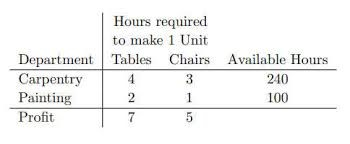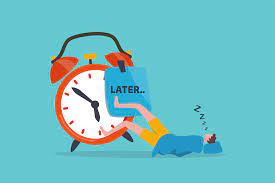# LINEAR PROGRAMMINGLinear programming, also abbreviated as LP, is a simple method we use to depict complicated real-world relationships by using a linear function. Therefore, we obtain the best outcome by minimizing or maximizing the objective function.

This is a follow up to the post about the optimization model. We will therefore be looking at some examples of linear programming. In other words, giving more explanation on the topic.

Linear Programming Formula

A linear programming problem will consist of decision variables, an objective function, constraints, and non-negative restrictions.

• The decision variables, x, and y decide the output of the LP problem and represent the final solution.
• The objective function, Z, is the linear function that needs to be optimized (maximized or minimized) to get the solution.
• The constraints are the restrictions that are imposed on the decision variables to limit their value.

Below is the general formula of a linear programming problem

Objective Function: Z = ax + by

Constraints: cx + dy ≤ e, fx + gy ≤ h. The inequalities can also be “≥”

Non-negative restrictions: x ≥ 0, y ≥ 0

How to Solve Linear Programming Problems?

The most important part of solving a linear programming problem is to first formulate the problem using the given data.

The steps to solve linear programming problems are given below:

Step 1: Identify the decision variables.

Step 2: Formulate the objective function. Check whether the function needs to be minimized or maximized.

Step 3: Write down the constraints.

Step 4: Ensure that the decision variables are greater than or equal to 0. (Non-negative restraint)

Step 5: Solve the linear programming problem. Use either the simplex or graphical method or Microsoft excel.

EXAMPLE

A company makes two products (X and Y) using two machines (A and B). To produce each unit of X, it requires 1 hour processing time on machine A and 1 hour 30 minutes processing time on machine B. And to produce each unit of Y, it requires 2 hours of processing time on machine A and 1 hour of processing time on machine B.

Also, the forecasted available processing time on machine A is 40 hours and on machine B is 35 hours.

The profit is N4 per product X N7 per product Y. Company policy is to maximize the combined sum of the units of X and the units of Y per production.

Formulate the problem of deciding how much of each product to make as a linear program.

SOLUTION

We need to follow the steps stated earlier in order to solve this problem.

Firstly, we are to identify the decision variables. The decision variables in this example are products X and Y.

Secondly, let us formulate the objective function. The objective function Z is to maximize profit on the quantity of X and Y.

Thus, Z=4X+7Y

Thirdly, write down the constraints.

In this example, the constraints are:

Machine A available processing time is 40 hours and for

Machine B available processing time is 35 hours

Then, formulate the problem:

Z=4X+7Y (Profit)

X+2Y<=40 (Machine A constraints)

1.5X+Y<=35 (Machine B constraints)

X,Y>=0 (Non-negativity)

The answer using the solver tool in excel is:

Therefore, the company needs to produce 15 units of product X and 12.5 units of Y to maximize profit.## Communicating in times of Crisis😊

in## The Psychology of Procrastination: Why We Delay and How to Overcome It”

in